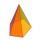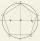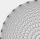# Nine-gon

Calculate the perimeter of a regular nonagon (9-gon) inscribed in a circle with a radius 13 cm.

Result

x =  80.03 cm

#### Solution:

$\theta = \dfrac{ 180^\circ }{ 9} = \dfrac{ \pi}{ 9} = 20 ^\circ \ \\ a = 2r \sin( \dfrac{ \pi}{ 9} ) = 8.89 \ cm \ \\ x = 9a = 80.03 \ \text { cm }$

Leave us a comment of this math problem and its solution (i.e. if it is still somewhat unclear...):Be the first to comment!## Next similar math problems:

1. Hexagon ACalculate area of regular hexagon inscribed in circle with radius r=9 cm.
2. Regular 5-gonCalculate area of the regular pentagon with side 7 cm.
3. Hexagonal pyramidCalculate the volume and the surface of a regular hexagonal pyramid with a base edge length of 3 cm and a height of 5 cm.
4. PentagonCalculate the area of regular pentagon, which diagonal is u=17.
5. 4side pyramidCalculate the volume and surface of 4 sides regular pyramid whose base edge is 4 cm long. The angle from the plane of the sidewall and base plane is 60 degrees.
6. Pentagonal pyramidCalculate the volume of a regular 5-side (pentaprism) pyramid ABCDEV; if |AB| = 7.7 cm and a plane ABV, ABC has angle 37 degrees.
7. n-gonWhat is the side length of the regular 5-gon inscribed in a circle of radius 12 cm?
8. PentagonWithin a regular pentagon ABCDE point P is such that the triangle is equilateral ABP. How big is the angle BCP? Make a sketch.
9. n-gon IIWhat is the side length of the regular 5-gon circumscribed circle of radius 11 cm?
10. TowerThe top of the tower is a regular hexagonal pyramid with base edge 8 meters long and a height 5 meters. How many m2 of the sheet is required to cover the top of the tower if we count 8% of the sheet waste?
11. Tetrahedral pyramidWhat is the surface of a regular tetrahedral (four-sided) pyramid if the base edge a=16 and height v=16?
12. HexagonThere is regular hexagon ABCDEF. If area of the triangle ABC is 22, what is area of the hexagon ABCDEF? I do not know how to solve it simply....
13. Octahedron - sumOn each wall of a regular octahedron is written one of the numbers 1, 2, 3, 4, 5, 6, 7 and 8, wherein on different sides are different numbers. For each wall John make the sum of the numbers written of three adjacent walls. Thus got eight sums, which also.
14. OctagonWe have a square with side 84 cm. We cut the corners to make his octagon. What will be the side of the octagon?
15. KitesBoys run kite on a cable of 68 meters long. What is the kite altitude, if the angle from the horizontal plane is 72°?
16. n-gonGabo draw n-gon, which angles are consecutive members of an arithmetic sequence. The smallest angle is 70° biggest 170°. How many sides have Gabo's n-gon?
17. Hexagon - MOThe picture shows the ABCD square, the EFGD square and the HIJD rectangle. Points J and G lie on the side CD and is true |DJ|